# VBA COUNTIF and COUNTIFS Functions

This tutorial will show you how to use the Excel COUNTIF and COUNTIFS functions in VBA

VBA does not have an equivalent of Excel’s COUNTIF or COUNTIFS Functions. Instead, you must call the Excel functions by using the WorkSheetFunction object.

## COUNTIF WorksheetFunction

The WorksheetFunction object can be used to call most of the Excel functions that are available within the Insert Function dialog box in Excel. The COUNTIF Function is one of them.

``````Sub TestCountIf()
Range("D10") = Application.WorksheetFunction.CountIf(Range("D2:D9"), ">5")
End Sub``````

The procedure above will only count the cells in Range(D2:D9) if they have a value of 5 or greater.  Notice that because you are using a greater than sign, the criteria greater than 5 needs to be within parenthesis.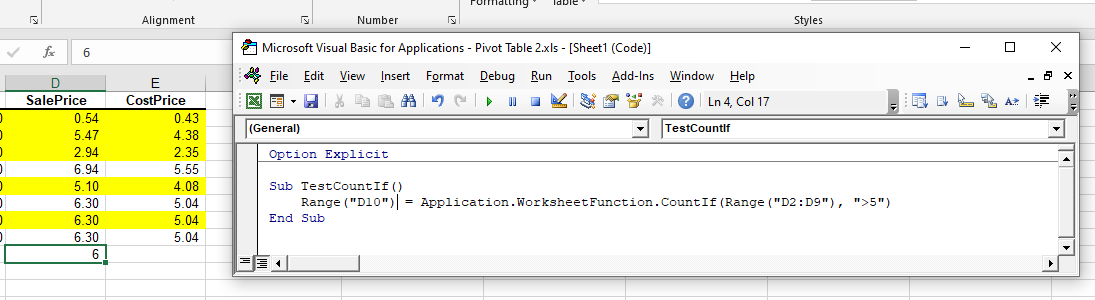## Assigning a COUNTIF result to a Variable

You may want to use the result of your formula elsewhere in code rather than writing it directly back to an Excel Range. If this is the case, you can assign the result to a variable to use later in your code.

``````Sub AssignSumIfVariable()
Dim result as Double
'Assign the variable
result = Application.WorksheetFunction.CountIf(Range("D2:D9"), ">5")
'Show the result
MsgBox "The count of cells with a value greater than 5 is " &  result
End Sub``````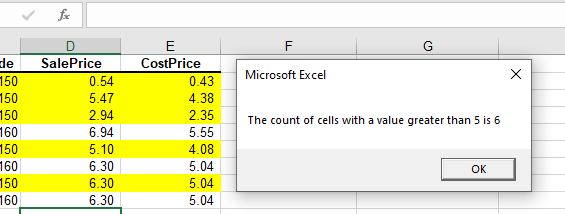## Using COUNTIFS

The COUNTIFS function is similar to the COUNTIF WorksheetFunction but it enables you to check for more than one criteria. In the example below, the formula will count up the number of cells in D2 to D9 where the Sale Price is greater than 6 AND the Cost Price is greater than 5.

``````Sub UsingCountIfs()
Range("D10") = WorksheetFunction.CountIfs(Range("C2:C9"), ">6", Range("E2:E9"), ">5")
End Sub``````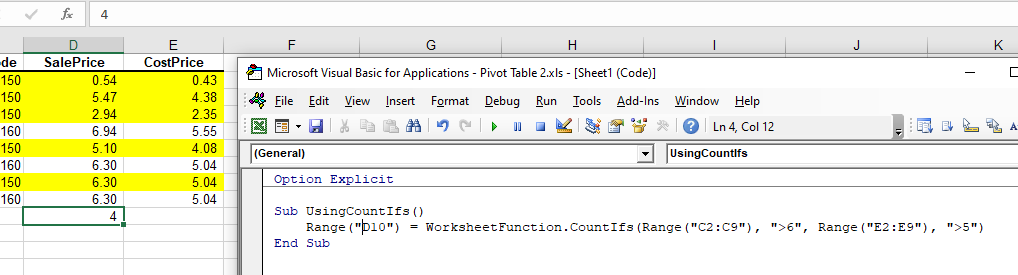## Using COUNTIF with a Range Object

You can assign a group of cells to the Range object, and then use that Range object with the WorksheetFunction object.

``````Sub TestCountIFRange()
Dim rngCount as Range
'assign the range of cells
Set rngCount = Range("D2:D9")
'use the range in the  formula
Range("D10") = WorksheetFunction.SUMIF(rngCount, ">5")
'release the range objects
Set rngCount = Nothing
End Sub``````

## Using COUNTIFS on Multiple Range Objects

Similarly, you can use COUNTIFS on multiple Range Objects.

``````Sub TestCountMultipleRanges()
Dim rngCriteria1 As Range
Dim rngCriteria2 as Range

'assign the range of cells
Set rngCriteria1= Range("D2:D9")
Set rngCriteria2 = Range("E2:E10")

'use the ranges in the formula
Range("D10") = WorksheetFunction.CountIfs(rngCriteria1, ">6", rngCriteria2, ">5")

'release the range objects
Set rngCriteria1 = Nothing
Set rngCriteria2 = Nothing
End Sub``````

## COUNTIF Formula

When you use the WorksheetFunction.COUNTIF to add a sum to a range in your worksheet, a static value is returned, not a flexible formula. This means that when your figures in Excel change, the value that has been returned by the WorksheetFunction will not change.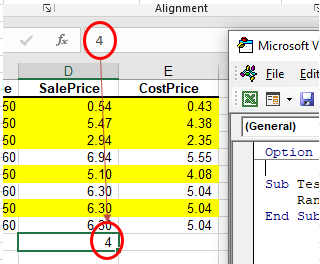In the example above, the procedure has counted the amount of cells with values in Range(D2:D9) where the Sale Price is greater than 6, and the result was put in D10. As you can see in the formula bar, this result is a figure and not a formula.

If any of the values change in Range(D2:D9),  the result in D10 will NOT change.

Instead of using the WorksheetFunction.SumIf, you can use VBA to apply a COUNTIF Function to a cell using the Formula or FormulaR1C1 methods.

Stop searching for VBA code online. Learn more about AutoMacro - A VBA Code Builder that allows beginners to code procedures from scratch with minimal coding knowledge and with many time-saving features for all users!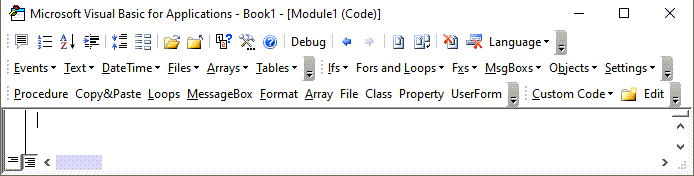### Formula Method

The formula method allows you to point specifically to a range of cells eg: D2:D9 as shown below.

``````Sub TestCountIf()
Range("D10").FormulaR1C1 ="=COUNTIF(D2:D9, "">5"")"
End Sub``````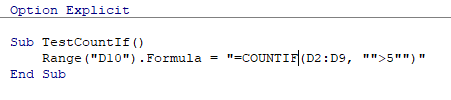### FormulaR1C1 Method

The FormulaR1C1 method is more flexible in that it does not restrict you to a set range of cells. The example below will give us the same answer as the one above.

``````Sub TestCountIf()
Range("D10").FormulaR1C1 = "=COUNTIF(R[-8]C:R[-1]C,"">5"")"
End Sub``````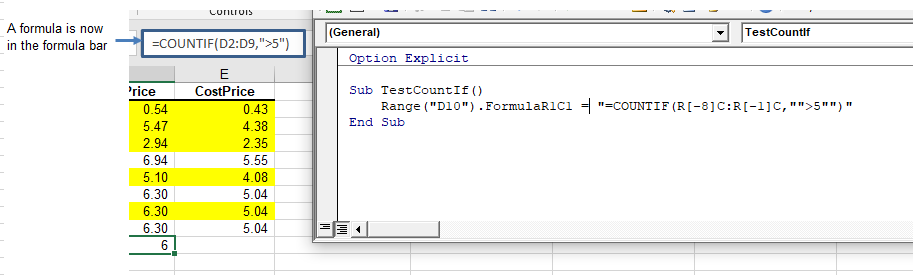However, to make the formula even more flexible, we could amend the code to look like this:

``````Sub TestCountIf()
ActiveCell.FormulaR1C1 = "=COUNTIF(R[-8]C:R[-1]C,"">5"")"
End Sub``````

Wherever you are in your worksheet, the formula will then count the cells that meet the criteria directly above it and place the answer into your ActiveCell. The Range inside the COUNTIF function has to be referred to using the Row (R) and Column (C) syntax.

Both these methods enable you to use Dynamic Excel formulas within VBA.

There will now be a formula in D10 instead of a value.